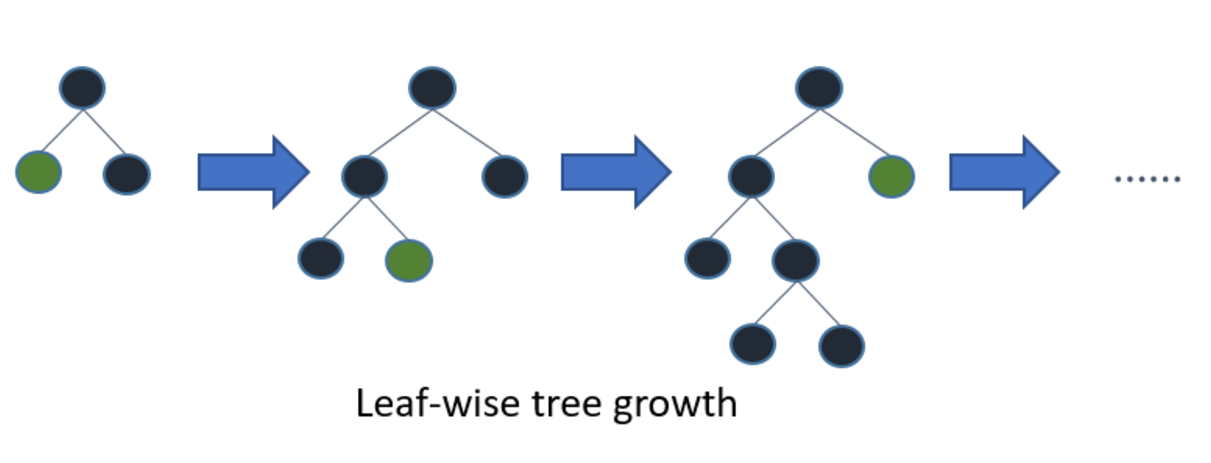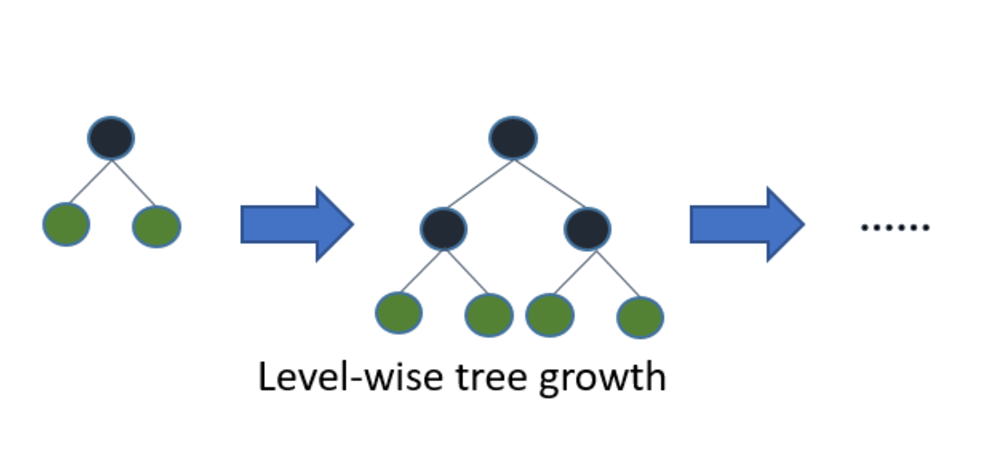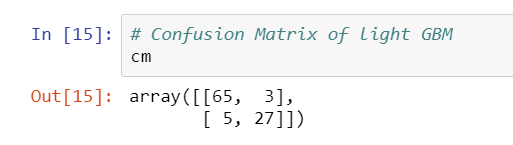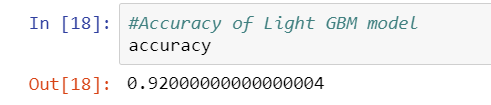# What is LightGBM, How to implement it? How to fine tune the parameters?

Hello,

Machine Learning is the fastest growing field in the world. Everyday there will be a launch of bunch of new algorithms, some of those fails and some achieve the peak of success. Today, I am touching one of the most successful machine learning algorithm, Light GBM.

What motivated me to write a blog on LightGBM?

While working on kaggle data science competition I came across multiple powerful algorithms. LightGBM is one of those. LightGBM is a relatively new algorithm and it doesn’t have a lot of reading resources on the internet except its documentation. It becomes difficult for a beginner to choose parameters from the long list given in the documentation. Simply to help new geeks, I am coming up with this beautiful blog.

I will try my best to keep this blog small and simple as adding hundreds of pages of irrelevant information will confuse you.

What is Light GBM?

Light GBM is a gradient boosting framework that uses tree based learning algorithm.

How it differs from other tree based algorithm?

Light GBM grows tree vertically while other algorithm grows trees horizontally meaning that Light GBM grows tree leaf-wise while other algorithm grows level-wise. It will choose the leaf with max delta loss to grow. When growing the same leaf, Leaf-wise algorithm can reduce more loss than a level-wise algorithm.

Below diagrams explain the implementation of LightGBM and other boosting algorithms.Explains how LightGBM worksHow other boosting algorithm works
Why Light GBM is gaining extreme popularity?

The size of data is increasing day by day and it is becoming difficult for traditional data science algorithms to give faster results. Light GBM is prefixed as ‘Light’ because of its high speed. Light GBM can handle the large size of data and takes lower memory to run. Another reason of why Light GBM is popular is because it focuses on accuracy of results. LGBM also supports GPU learning and thus data scientists are widely using LGBM for data science application development.

Can we use Light GBM everywhere?

No, it is not advisable to use LGBM on small datasets. Light GBM is sensitive to overfitting and can easily overfit small data. Their is no threshold on the number of rows but my experience suggests me to use it only for data with 10,000+ rows.

We briefly discussed the concept of Light GBM, now what about it’s implementation?

Implementation of Light GBM is easy, the only complicated thing is parameter tuning. Light GBM covers more than 100 parameters but don’t worry, you don’t need to learn all.

It is very important for an implementer to know atleast some basic parameters of Light GBM. If you carefully go through following parameters of LGBM, I bet you will find this powerful algorithm a piece of cake.

Let’s start discussing parameters.

### Parameters

Control Parameters

max_depth: It describes the maximum depth of tree. This parameter is used to handle model overfitting. Any time you feel that your model is overfitted, my first advice will be to lower max_depth.

min_data_in_leaf: It is the minimum number of the records a leaf may have. The default value is 20, optimum value. It is also used to deal over fitting

feature_fraction: Used when your boosting(discussed later) is random forest. 0.8 feature fraction means LightGBM will select 80% of parameters randomly in each iteration for building trees.

bagging_fraction: specifies the fraction of data to be used for each iteration and is generally used to speed up the training and avoid overfitting.

early_stopping_round: This parameter can help you speed up your analysis. Model will stop training if one metric of one validation data doesn’t improve in last early_stopping_round rounds. This will reduce excessive iterations.

lambda: lambda specifies regularization. Typical value ranges from 0 to 1.

min_gain_to_split: This parameter will describe the minimum gain to make a split. It can used to control number of useful splits in tree.

max_cat_group: When the number of category is large, finding the split point on it is easily over-fitting. So LightGBM merges them into ‘max_cat_group’ groups, and finds the split points on the group boundaries, default:64

Core Parameters

Task: It specifies the task you want to perform on data. It may be either train or predict.

application: This is the most important parameter and specifies the application of your model, whether it is a regression problem or classification problem. LightGBM will by default consider model as a regression model.

• regression: for regression
• binary: for binary classification
• multiclass: for multiclass classification problem

boosting: defines the type of algorithm you want to run, default=gdbt

• gbdt: traditional Gradient Boosting Decision Tree
• rf: random forest
• dart: Dropouts meet Multiple Additive Regression Trees
• goss: Gradient-based One-Side Sampling

num_boost_round: Number of boosting iterations, typically 100+

learning_rate: This determines the impact of each tree on the final outcome. GBM works by starting with an initial estimate which is updated using the output of each tree. The learning parameter controls the magnitude of this change in the estimates. Typical values: 0.1, 0.001, 0.003…

num_leaves: number of leaves in full tree, default: 31

device: default: cpu, can also pass gpu

Metric parameter

metric: again one of the important parameter as it specifies loss for model building. Below are few general losses for regression and classification.

• mae: mean absolute error
• mse: mean squared error
• binary_logloss: loss for binary classification
• multi_logloss: loss for multi classification
IO parameter

max_bin: it denotes the maximum number of bin that feature value will bucket in.

categorical_feature: It denotes the index of categorical features. If categorical_features=0,1,2 then column 0, column 1 and column 2 are categorical variables.

ignore_column: same as categorical_features just instead of considering specific columns as categorical, it will completely ignore them.

save_binary: If you are really dealing with the memory size of your data file then specify this parameter as ‘True’. Specifying parameter true will save the dataset to binary file, this binary file will speed your data reading time for the next time.

Knowing and using above parameters will definitely help you implement the model. Remember I said that implementation of LightGBM is easy but parameter tuning is difficult. So let’s first start with implementation and then I will give idea about the parameter tuning.

### Implementation

Installating LGBM:

Installing LightGBM is a crucial task. I found this as the best resource which will guide you in LightGBM installation.

I am using Anaconda and installing LightGBM on anaconda is a clinch. Just run the following command on your Anaconda command prompt and whoosh, LightGBM is on your PC.

`conda install -c conda-forge lightgbm`

Dataset:

This data is very small just 400 rows and 5 columns (specially used for learning purpose). This is a classification problem where we have to predict whether a customer will buy the product from advertise given on the website. I am not explaining dataset as dataset is self-explanatory. You can download dataset from my drive.

Note: The dataset is clean and has no missing value. The main aim behind choosing this much smaller data is to keep the things simpler and understandable.

I am assuming that you all know basics of python. Go through data preprocessing steps, they are fairly easy but if you have any doubt then ask me in the comment, I will get back to you asap.

Data preprocessing:

`import numpy as npimport matplotlib.pyplot as pltimport pandas as pd`
`# Importing the datasetdataset = pd.read_csv('...input\\Social_Network_Ads.csv')X = dataset.iloc[:, [2, 3]].valuesy = dataset.iloc[:, 4].values`
`# Splitting the dataset into the Training set and Test setfrom sklearn.cross_validation import train_test_splitx_train, x_test, y_train, y_test = train_test_split(X, y, test_size = 0.25, random_state = 0)`
`# Feature Scalingfrom sklearn.preprocessing import StandardScalersc = StandardScaler()x_train = sc.fit_transform(x_train)x_test = sc.transform(x_test)`

Model building and training:

We need to convert our training data into LightGBM dataset format(this is mandatory for LightGBM training).

After creating a converting dataset, I created a python dictionary with parameters and their values. Accuracy of your model totally depends on the values you provide to parameters.

In the end block of code, I simply trained model with 100 iterations.

`import lightgbm as lgb`
`d_train = lgb.Dataset(x_train, label=y_train)`
`params = {}params['learning_rate'] = 0.003params['boosting_type'] = 'gbdt'params['objective'] = 'binary'params['metric'] = 'binary_logloss'params['sub_feature'] = 0.5params['num_leaves'] = 10params['min_data'] = 50params['max_depth'] = 10`
`clf = lgb.train(params, d_train, 100)`

Few things to notice in parameters:

• Used ‘binary’ as objective(remember this is classification problem)
• Used ‘binary_logloss’ as metric(same reason, binary classification problem)
• ‘num_leaves’=10 (as it is small data)
• ‘boosting type’ is gbdt, we are implementing gradient boosting(you can try random forest)

Model prediction:

we just need to write a line for predictions.

Output will be a list of probabilities. I converted probabilities to binary prediction keeping threshold=0.5

`#Predictiony_pred=clf.predict(x_test)`
`#convert into binary values`
`for i in range(0,99):    if y_pred[i]>=.5:       # setting threshold to .5       y_pred[i]=1    else:         y_pred[i]=0`

### Results:

We can check results either using confusion matrix or directly calculating accuracy

Code:

`#Confusion matrix`
`from sklearn.metrics import confusion_matrixcm = confusion_matrix(y_test, y_pred)`
`#Accuracy`
`from sklearn.metrics import accuracy_scoreaccuracy=accuracy_score(y_pred,y_test)`

Screenshots of result:Confusion MatrixAccuracy Score

Many of you must be thinking that I used smaller dataset and still my model has 92% accuracy. Why there is no overfitting? The simple reason is I fine tuned model parameters.

So now let’s jump into parameter fine tuning.

### Parameter Tuning:

Data scientists always struggle in deciding when to use which parameter? and what should be the ideal value of that parameter?

Following set of practices can be used to improve your model efficiency.

1. num_leaves: This is the main parameter to control the complexity of the tree model. Ideally, the value of num_leaves should be less than or equal to 2^(max_depth). Value more than this will result in overfitting.
2. min_data_in_leaf: Setting it to a large value can avoid growing too deep a tree, but may cause under-fitting. In practice, setting it to hundreds or thousands is enough for a large dataset.
3. max_depth: You also can use max_depth to limit the tree depth explicitly.

For Faster Speed:

• Use bagging by setting `bagging_fraction` and `bagging_freq`
• Use feature sub-sampling by setting `feature_fraction`
• Use small `max_bin`
• Use `save_binary` to speed up data loading in future learning
• Use parallel learning, refer to parallel learning guide.

For better accuracy:

• Use large `max_bin` (may be slower)
• Use small `learning_rate` with large `num_iterations`
• Use large `num_leaves`(may cause over-fitting)
• Use bigger training data
• Try `dart`
• Try to use categorical feature directly

To deal with over-fitting:

• Use small `max_bin`
• Use small `num_leaves`
• Use `min_data_in_leaf` and `min_sum_hessian_in_leaf`
• Use bagging by set `bagging_fraction` and `bagging_freq`
• Use feature sub-sampling by set `feature_fraction`
• Use bigger training data
• Try `lambda_l1`, `lambda_l2` and `min_gain_to_split` to regularization
• Try `max_depth` to avoid growing deep tree

### Conclusion:

I implemented LightGBM on multiple datasets and found that its accuracy challenged other boosting algorithms. From my experience, I will always recommend you to try this algorithm at least once.

I hope you guys enjoyed this blog and it was useful to all. I would request you you to give suggestion which will help to improve this blog.

Source: Microsoft LightGBM Documentation

Thanks,

Pushkar Mandot